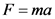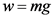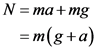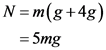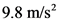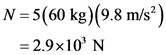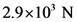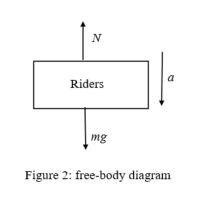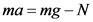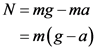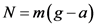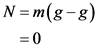# What is a 60 kg rider’s apparent weight during the launch?

Riders on the Power Tower are launched skyward with an
acceleration of 4g, after which they experience a period of free
fall. (Figure 1)Part A Part A What is a 60 kg rider’s apparent weight during the launch? Express your answer to two significant figures and
include the appropriate units. Express your answer to two significant figures and
include the appropriate units. w = w Part B Part B What is a 60 kg rider’s apparent weight during the period of
free fall? Express your answer to two significant figures and
include the appropriate units. Express your answer to two significant figures and
include the appropriate units. w =
w

General guidance

Concepts and reason
The concept required to solve this question is Newton’s Second law, weight, normal force, and free fall motion.
Initially, the riders are accelerated upward. So, find the force acting on the riders using Newton’s second law. Then, the riders experience free fall and so the riders falls with an acceleration that is equal to acceleration due to gravity.

Fundamentals

The Newton’s second law states that the force is equal to the product of the mass and the acceleration.
The expression of the force is,

Here, F is the force, m is the mass, and a is the acceleration.
The weight of the rider is,

Here, g is the acceleration due to gravity.

Step-by-step

Step 1 of 2

(A)
The following figure shows the free body diagram of the system:

Here, N is the normal force, m is the mass, and g is the acceleration due to gravity, and a is the acceleration.
Refer the above diagram and write the equation of the motion.
The equation of motion using the Newton’s second law when the riders are accelerated upwards is,

Rearrange the equation for N.

The apparent weight during the launch is,

Substitute 4g for a.

Substitute 60 kg for m and for g.

Part A
The apparent weight during the launch is.

The weight of the rider is acting downwards and the normal force exerted by the seats acting upwards. As the acceleration of rider is upward, the net force is also acting upwards.

The acceleration of riders is acting upwards. Do not consider it as downwards.

Step 2 of 2

(B)
The following figure shows the free body diagram of the system:

Here, N is the normal force, m is the mass, and g is the acceleration due to gravity, and a is the acceleration.
Refer the above diagram and write the equation of the motion.
The equation of motion using the Newton’s second law when the riders are accelerated downwards is,

Rearrange the equation.

The apparent weight during the fall is,

Substitute g for a in the above equation as the rider is falling under free fall.

Part B
The apparent weight during the fall is 0 N.

In free fall motion, the objects accelerate with an acceleration that is equal to acceleration due to gravity. Hence, the acceleration a is replaced with acceleration due to gravity g in the apparent weight equation.
In the given scenario, the normal force on the rider gives the apparent weight of the rider.

Part A
The apparent weight during the launch is.

Part B
The apparent weight during the fall is 0 N.

Part A
The apparent weight during the launch is.

Part B
The apparent weight during the fall is 0 N.

F=ma
w=mg
Riders mg Figure 1: free-body diagram
ma=N-mg
N=ma+mg = m(g+a)
N=m(g+a)
N=m(g+48) = 5mg
9.8 m/s?
N = 5(60 kg)(9.8 m/s) = 2.9×10′ N
Riders mg Figure 2: free-body diagram
ma = mg -N
N = mg -ma = m(g-a)
N=m(g-a)
N = m(8-8) =0
2.9×10′ N
2.9×10′ N